Courses

# Test: Previous Year Questions: States Of Matter

## 14 Questions MCQ Test Chemistry Class 11 | Test: Previous Year Questions: States Of Matter

Description
This mock test of Test: Previous Year Questions: States Of Matter for JEE helps you for every JEE entrance exam. This contains 14 Multiple Choice Questions for JEE Test: Previous Year Questions: States Of Matter (mcq) to study with solutions a complete question bank. The solved questions answers in this Test: Previous Year Questions: States Of Matter quiz give you a good mix of easy questions and tough questions. JEE students definitely take this Test: Previous Year Questions: States Of Matter exercise for a better result in the exam. You can find other Test: Previous Year Questions: States Of Matter extra questions, long questions & short questions for JEE on EduRev as well by searching above.
QUESTION: 1

### According to the kinetic theory of gases, in an ideal gas, between two successive collisions a gas molecule travels –    [AIEEE-2003]

Solution:

According to kinetic theory the gas molecules are in a state of constant rapid motion in all possible directions colloiding in a random manner with one another and with the walls of the container and between two successive collisions molecules travel in a straight line path but show haphazard motion due to collisions.

QUESTION: 2

### As the temperature is raised from 20°C to 40°C, the average kinetic energy of neon atoms changes by a factor of which of the following ? [AIEEE-2004]

Solution:

Average kinetic energy ∝ Temperature in Kelvin

(KE40) / (KE20) = (3/2 nR * 313)/(3/2 nR*293)

KE40 / KE20 = T2/T1 = 40 + 273 / 20 + 273  = 313/293

QUESTION: 3

### In van der Waals equation of state of the gas law, the constant `b' is a measure of - [AIEEE-2004]

Solution:

The correct answer is Option C.

In van der Waals equation of state of the gas law, the constant b is a measure of the volume occupied by the molecules.

It gives the effective size of the gas molecules. The greater value of b indicates a larger size of the molecules and smaller compressible volume.

QUESTION: 4

Which one of the following statements is NOT true about the effect of an increase in temperature on the distribution of molecular speeds in a gas ?

[AIEEE-2005]

Solution:

Most probable velocity increase and fraction of molecule possessing most probable velocity decrease.

QUESTION: 5

Equal masses of methane and oxygen are mixed in an empty container at 25ºC. The fraction of the total pressure exerted by oxygen is –

[AIEEE-2007]

Solution:

Let the mass of methane and oxygen be m gm.
Mole fraction of oxygen, xO2 = m/32/(m/32 +m/16) = 1/3
Let the total pressure be P.
Partial pressure of O2, pO2 = P × xO2 = P x 1/3 = P/3

QUESTION: 6

The no. of moles per litre in the equation PV = nRT is expressed by -

[AIEEE-2002]

Solution:

Number of moles per litre can be represented as n/V so if we shift RT to the left side and V to the right side then we can get n/V that is our number of moles per litre is equal to p/RT and so option a is correct.

QUESTION: 7

The correct value of R is -

[AIEEE-2002]

Solution:

The different values of R are as follow:-
8.314 J mol-1 K-1, 8.314×107 erg mol-1 K-1, 0.0821 atm-lit mol-1 K-1 or 2 cal mol-1 K-1

QUESTION: 8

If 10-4 dm3 of water is introduced into a 1.0 dm3 flask at 300 K, how many moles of water are in in the vapour phase when equilibrium is established ?

(Given : Vapour pressure of H2O at 300 is 3170 pa; R = 8.314 JK-1 mol)

[AIEEE -2010]

Solution:

The volume occupied by water molecules in vapour phase is (1×10−4) dm3, that is approximately (1×10−3) m3.
pvapV = nH2O mol
3170 × 1 × 10−3 = nH2O × 8.314 × 300K
nH2O = 3170 × 1 × 10−3 / 8.314 × 300
= 1.27 × 10−3 mol

QUESTION: 9

`a' and `b' are Vander Waals' constant for gases. Chlorine is more easily liquefied than ethane because :

[AIEEE - 2011]

Solution:

a and b are the van der Waals constant for gases where ‘a’ means the force of attraction while ‘b’ is the effective size of the molecule. Chlorine is more easily liquefied than Ethane so ‘a’  of chlorine will must be greater than a value of Ethane. Also, Chlorine is more easily liquefied then the ‘b’ of chlorine should be less than ‘b’ of Ethane. For smaller size we have more attraction and so the liquefaction will be easy.

QUESTION: 10

When r, P and M represent rate of diffusion, pressure and molecular mass, respectively, then the ratio of the rates of diffusion (rA/rB) of two gases A and B, is given as -

[AIEEE - 2011]

Solution:

The correct answer is option A
Rate of diffusion: r     α    p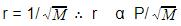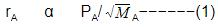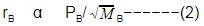rA/rB = pA/pB.(MB/MA)1/2
Hence, pBpA (MB/MA)1/2 is the answer.

QUESTION: 11

The molecular velocity of any gas is -

[AIEEE - 2011]

Solution:

The average kinetic energy of a gas particle is directly proportional to the temperature. An increase in temperature increases the speed in which the gas molecules move. All gases at a given temperature have the same average kinetic energy. Lighter gas molecules move faster than heavier molecules.

QUESTION: 12

The compressibility factor for a real gas at high pressure is –

[AIEEE-2012]

Solution: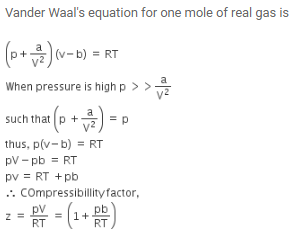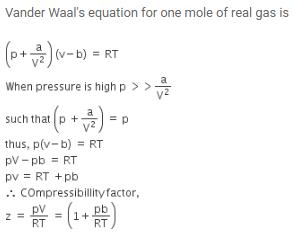QUESTION: 13

For gaseous state, if most probable speed is denoted by C*, average speed by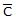and mean square speed by C, then for a large number of molecules the ratios of these speeds are :

[Jee(Main) 2013, 3/120]

Solution: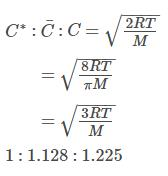QUESTION: 14

A gaseous hydrocarbon gives upon combustion 0.72 g of water and 3.08 g. of CO2. The empirical formula of the Hydrocarbon is :

[Jee(Main) 2013, 3/120]

Solution:

General equation for combustion of hydrocarbon:
CxHy + (x+ y/4)O2 → xCO2 + (y/2)H2O
Number of moles of CO2 produced = 3.08/44 = 0.07
Number of moles of H2O produced = 0.72/18 = 0.04
SO, x / (y/2) = 0.07/0.04 =  7/4
The formula of hydrocarbon is C7H8